Next: Conservation Laws Up: Planetary Motion Previous: Kepler's Laws

Newtonian Gravity

The force which maintains the Planets in orbit around the Sun is called gravity, and was first correctly described by Isaac Newton (in 1687). According to Newton, any two point mass objects (or spherically symmetric objects of finite extent) exert a force of attraction on one another. This force points along the line of centers joining the objects, is directly proportional to the product of the objects' masses, and inversely proportional to the square of the distance between them. Suppose that the first object is the Sun, which is of mass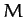, and is located at the origin of our coordinate system. Let the second object be some planet, of mass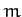, which is located at position vector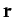. The gravitational force exerted on the planet by the Sun is thus written(210)

The constant of proportionality,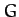, is called the gravitational constant, and takes the value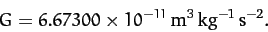(211)

An equal and opposite force to (210) acts on the Sun. However, we shall assume that the Sun is so much more massive than the planet in question that this force does not cause the Sun's position to shift appreciably. Hence, the Sun will always remain at the origin of our coordinate system. Likewise, we shall neglect the gravitational forces exerted on our planet by the other planets in the Solar System, compared to the much larger gravitational force exerted by the Sun.

Incidentally, there is something rather curious about Equation (210). According to this law, the gravitational force acting on an object is directly proportional to its inertial mass. But why should inertia be related to the force of gravity? After all, inertia measures the reluctance of a given body to deviate from its preferred state of uniform motion in a straight-line, in response to some external force. What has this got to do with gravitational attraction? This question perplexed physicists for many years, and was only answered when Albert Einstein published his general theory of relativity in 1916. According to Einstein, inertial mass acts as a sort of gravitational charge since it impossible to distinguish an acceleration produced by a gravitational field from an apparent acceleration generated by observing in a non-inertial reference frame. The assumption that these two types of acceleration are indistinguishable leads directly to all of the strange predictions of general relativity: e.g., clocks in different gravitational potentials run at different rates, mass bends space, etc.

According to Equation (210), and Newton's second law, the equation of motion of our planet takes the form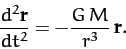(212)

Note that the planetary mass,, has canceled out on both sides of the above equation.Next: Conservation Laws Up: Planetary Motion Previous: Kepler's Laws
Richard Fitzpatrick 2011-03-31